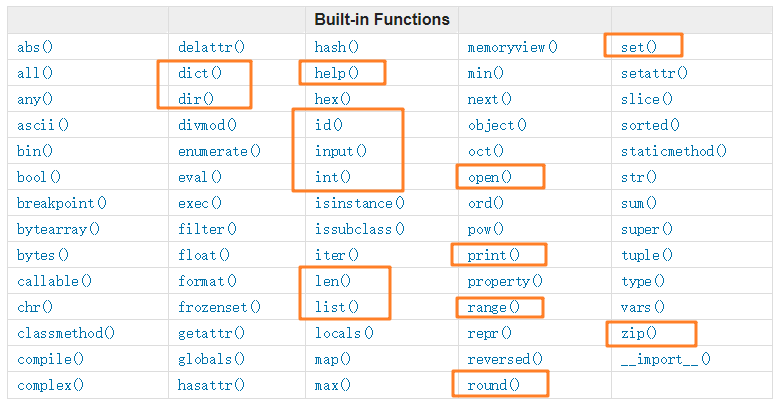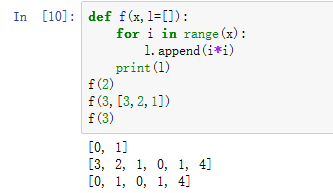## 今天的面试题

### 第1题：1行代码实现1到100的和？

Python常见的内置函数有https://docs.python.org/3/library/functions.html

sum(iterable[, start])

1. iterable – 可迭代对象，如：列表、元组、集合。
2. start – 指定相加的参数，如果没有设置这个值，默认为0。

``````sum([1,2,3]) # 结果为6
sum([1,2,3],5) # 结果为11
``````

python一行代码如何实现1~100的和

range(start, stop[, step])

1. start: 计数从 start 开始。默认是从 0 开始。例如range（5）等价于range（0， 5）;
2. stop: 计数到 stop 结束，但不包括 stop。例如：range（0， 5） 是[0, 1, 2, 3, 4]没有5
3. step：步长，默认为1。例如：range（0， 5） 等价于 range(0, 5, 1)

``````sum(range(1,101))
``````

### 第2题：如何使用Python输出一个[斐波那契数列]Fibonacci

100以内的斐波那契数列

``````x=1
y=1
print(x,end=" ")
print(y,end=" ")
while(True):
z=x+y
x=y
y=z
if(z>100):   #当z>100的时候，终止循环
break

print(z,end=" ")

``````

``````#递归
def fibo(n):

if n <= 1:
return n
else:
return (fibo(n - 1) + fibo(n - 2))

m = int(input("打印前多少项?"))
if m <= 0:
print("请输入正整数！")
else:
print("fibo:")
for i in range(1,m):
print(fibo(i))

``````

``````def fibo(max):
n, a, b = 0, 0, 1
while n < max:
yield b
a, b = b, a + b
n = n + 1  # 退出标识
for n in fibo(5):
print (n)
``````

time.clock()说明

• cpu 的运行机制：cpu是多任务的，例如在多进程的执行过程中，一段时间内会有对各进程被处理。一个进程从从开始到结束其实是在这期间的一些列时间片断上断断续续执行的。所以这就引出了程序执行的cpu时间（该程序单纯在cpu上运行所需时间）和墙上时钟wall time。
• time.time()是统计的wall time(即墙上时钟)，也就是系统时钟的时间戳（1970纪元后经过的浮点秒数）。所以两次调用的时间差即为系统经过的总时间。
• time.clock()是统计cpu时间 的工具，这在统计某一程序或函数的执行速度最为合适。两次调用time.clock()函数的插值即为程序运行的cpu时间。
``````import time
def fibo(max):
n, a, b = 0, 0, 1
while n < max:
yield b
a, b = b, a + b
n = n + 1
t1 = time.clock()
for n in fibo(100):
print (n)

t2 = time.clock()

print(t2-t1)
``````

``````import time
def fibo(n):

result_list = []
a, b = 0, 1
while n > 0:
result_list.append(b)
a, b = b, a + b
n -= 1
return result_list

t1 = time.clock()
print(fibo(1000))
t2 = time.clock()
print(t2-t1)
``````

https://blog.csdn.net/chichu261/article/details/83589767

### 第3题：列出几个python标准库

Python标准库(standard library)。

1. os模块
2. re模块
3. pickle 模块
4. datetime模块
5. time模块
6. math模块

### 第4题：下面Python代码的运行结果是？

``````
def f(x,l=[]):
for i in range(x):
l.append(i*i)
print(l)
f(2)
f(3,[3,2,1])
f(3)

``````
• f(2)
``````def f(2,l=[]):
for i in range(2): # i=0,1
l.append(i*i) # [0,1]
print(l)
``````
• f(3,[3,2,1])
``````def f(3,l=[3,2,1]):
for i in range(3): # i=0,1,2
l.append(i*i) # [3,2,1,0,1,4]
print(l)
``````
• f(3)
``````def f(3,l=[]):
for i in range(3): # i=0,1,2
l.append(i*i) # [0,1,4] ???对吗？
print(l)
``````

`f(3,[3,2,1])` 将l进行了重新赋值。但是第三次调用函数使用的依旧是第一次的l,所以避免踩坑哦~~~~

f(3)运行的正确结果是`[0,1,0,1,4]`### 第5题：python实现列表去重的方法？

``````my_list = [1,1,2,2,3,3,5,6,7,88]
my_set = set(my_list)
my_list = [x for x in my_set]
my_list
``````

``````ids = [1,1,2,2,3,3,5,6,7,88]
news_ids = []
for id in ids:
if id not in news_ids:
news_ids.append(id)
print(news_ids)

``````

``````my_list=[1,1,2,2,3,3,5,6,7,88]
d = {}.fromkeys(my_list)
print(d.keys())
``````

## 今天的5道面试题答题完毕

nice girl 今天的5道面试题回答完毕~不知道你学到了多少，下篇博客见哦！